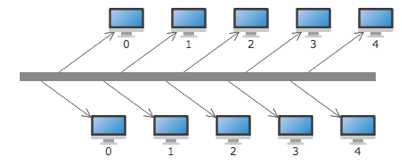Light High Efficiency Graph Component

# Bus Effect

Bus layout is a typical structure in the topological graph. Bus is a public messaging trunk for transmitting information among each functional part of computer. The bus and devices connected to it form a topological structure, which is called bus topological graph. In Qunee, the Bus element type is defined. But the edge has to be set for getting the bus effect.

The edge angle property is defined in Qunee. In comination with bus element type, edge effect in certain direction can be realized

Edge#angle - strike angle of edge

Bus example

``````var graph = new Q.Graph("canvas");
var bus = new Q.Bus();
bus.moveTo(-200, 0);
bus.lineTo(200, 0);
bus.setStyle(Q.Styles.SHAPE_STROKE, 10);
for(var i = 0; i < 5; i++){
var node = graph.createNode("" + i, -100 + i * 70, 60);
var edge = graph.createEdge(bus, node);
edge.angle = Math.PI * 0.2;
node = graph.createNode("" + i, -80 + i * 70, -60);
edge = graph.createEdge(bus, node);
edge.angle = -Math.PI * 0.2;
}
``````

Operation effect